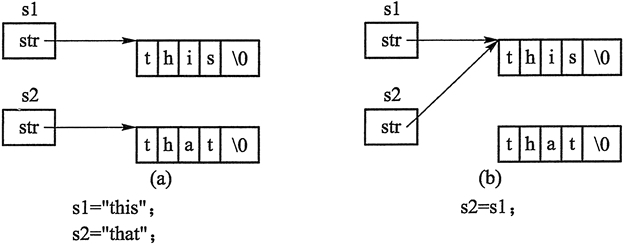## C++深拷贝和浅拷贝（C++深复制和浅复制）

• 内容
• 评论
• 相关

```#include <iostream>
#include <cstring>
using namespace std;
class String {
private:
char * str;
public:
String() :str(NULL) { }
const char * c_str() const { return str; };
String & operator = (const char * s);
~String();
};
String & String::operator = (const char * s)
//重载"="以使得 obj = "hello"能够成立
{
if (str)
delete[] str;
if (s) {  //s不为NULL才会执行拷贝
str = new char[strlen(s) + 1];
strcpy(str, s);
}
else
str = NULL;
return *this;
}
String::~String()
{
if (str)
delete[] str;
};
int main()
{
String s;
s = "Good Luck,"; //等价于 s.operator=("Good Luck,");
cout << s.c_str() << endl;
// String s2 = "hello!";   //这条语句要是不注释掉就会出错
s = "Shenzhou 8!"; //等价于 s.operator=("Shenzhou 8!");
cout << s.c_str() << endl;
return 0;
}```

```String s1, s2;
s1 = "this";
s2 = "that";
s2 = s1;```0条评论